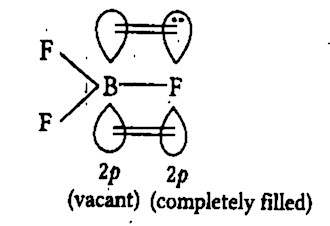Home/Class 11/Chemistry/

## QuestionChemistryClass 11

Account for the following observations. Though fluorine is more electronegative than chlorine yet $$BF_{3}$$ is weaker Lewis acid than$$BCl_3$$.

Lewis acids are generally electron-deficient species. In the case of $$BF_3$$, the boron has vacant $$2p$$-orbital and fluorine has one completely filled $$2p$$ orbital. The electron from filled orbital goes to vacant orbital because both of these orbitals belong to the same energy level therefore, they can overlap effectively and form a $$p\pi- \ p\pi$$ double bond. This type of bond formation is known as back bonding as shown:On the other hand, in the case of $$BCl_3$$, there is no effective overlapping of $$2p-$$ orbital of boron and $$3p-$$ orbital of chlorine. Hence, $$BCl_3$$ is more electron deficient species than $$BF_3$$. That's why $$BF_{3}$$ is a weaker Lewis acid than $$BCl_{3}$$.